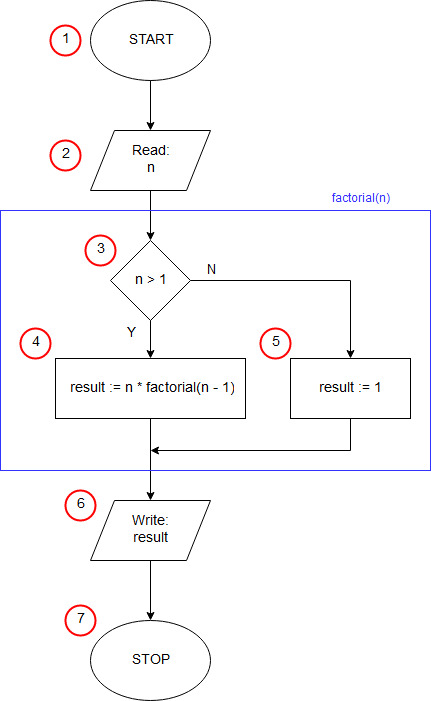# Factorial in JavaScript - recursiveIn the 1st line of the program, we inform the browser that our document was written in HTML (more precisely, it is an HTML 5 header). Line 2 contains the <html> tag that begins the HTML code. The 3rd line starts the <body> section of our document, containing the elements that are to appear on the page. In our example, the <body> section contains only a script written in JavaScript that implements the algorithm for solving a linear equation. The <script> tag from line 4 informs about the start of the script - we can treat it as the starting block (1) of our algorithm. The content of the script is contained in lines 6 to 14.

In line 6 we declare a unary function called factorial. Between braces { from the end of line 6 and } from line 11 there is a function body - a set of instructions executed by the program after calling this function.

In line 7 we check if n is greater than 1 - block (3) of the algorithm. If so, in line 8 we specify the result of the function as n multiplied by the value of the factorial function (the same factorial function we are currently defining) for n minus 1 (4). At this point, the factorial function calls itself. We call this a recursive function. If n is not greater than 1, then in line 10 we set the result of the function equal to 1 (recursion termination condition) - block (5).

In line 13, we declare a variable n using the var keyword, while the assignment operator ("=") initializes this variable with the value entered by the user using the prompt() statement - block (2) of the algorithm. prompt - JavaScript standard function - displays a text input pop-up on the screen. The first argument of the function specifies the text displayed as a prompt, the second is the default value entered in the edit field. In our case, we're displaying "n:" as the prompt, and we're not prompting the user with any default text (the second argument is the empty text ""). As a result, the prompt() function returns the text entered by the user into the edit field and then confirmed with the OK button.

In line 14, using the alert() function, we print the value of variable n, the text "!=" and finally the result of calling the function factorial(n) - block (6) of the algorithm.

The </script> tag from line 16 terminates the program (end of algorithm block (9)).

Then, in lines 17 and 18, we close the </body> and </html> sections.